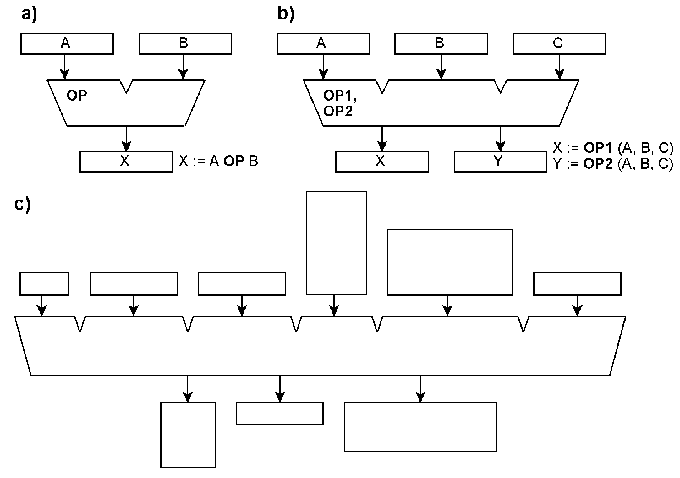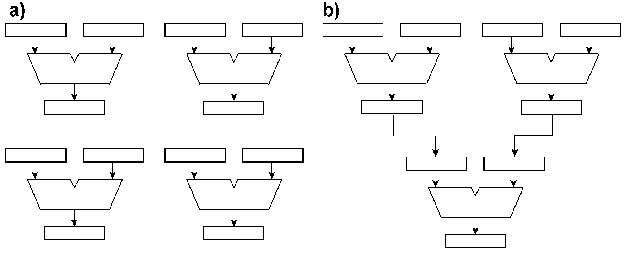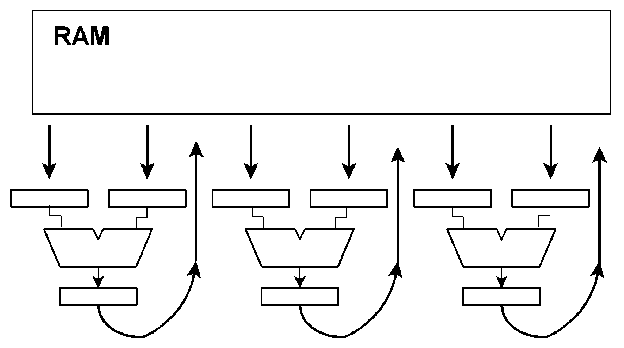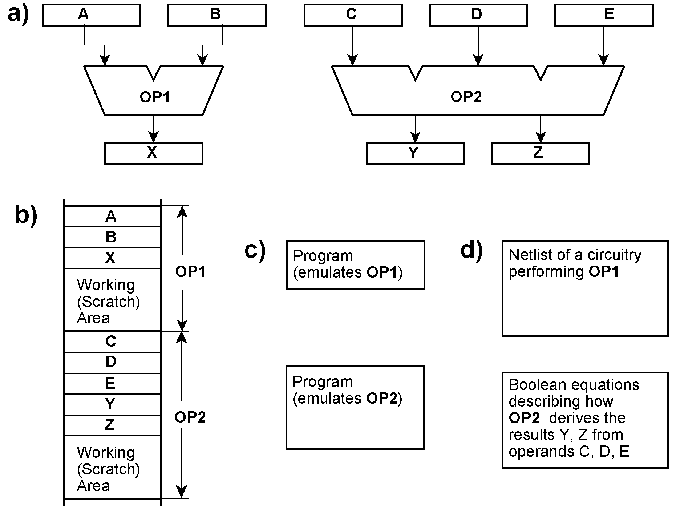Homepage

# The ReAl Computer Architecture

#### Theory

 News

Basic Resources

Fig. 1 illustrates the term of register transfer level (RTL).  Principally, each digital information processing system consists of memory means (flip-flops, registers, memory cells) and combinational networks. Its function is determined completely by the memory means RG and by the Boolean equations that describe the combinational networks CN.  In the following, the resources can therefore be illustrated by simple register transfer level (RTL) diagrams. RTL diagrams and Boolean equations that describe the functional units of conventional computers can be found in numerous textbooks, hardware manuals and the like.Fig. 1  Principal illustration of the register transfer level (RTL). RG = memory means (registers), CN = combinational networks.

Fig. 2 shows register transfer level diagrams of simple resources, comprising registers and combinational networks. For example, elementary resources (fig. 1.6a) generate a single result (X) from two operators (A, B):

X := A OP B   or    X := OP (A, b)

Most of the processing instructions of typical general-purpose computers correspond to this scheme (the differences lie primarily in the way how the operands are delivered and how the result is assigned). The well-known arithmetic logic units (ALUs) can be viewed as examples of such elementary resources.

General-purpose computers know only a few elementary data types, for example, integers, floating point numbers, characters, strings and so on. The operation  unit usually processes only data of some particular types (for example, integers or floating point numbers).  In case of elementary operations, the operands and the results have the same format.

ReAl resources have no such limitations.  A resource can create from an arbitrary number of operands any number of results, wherein the operands and the results may belong to any data type or data format (fig. 2b, c).  There is also no limitation to elementary data types.  The data types can be as complex as desired (bit and character strings, arrays, heterogeneous structures (records) and the like).Fig. 2  Some basic resources.

The typical general-purpose computer executes one instruction at a time. Hence a single processing resource is sufficient.  It is self-evident to increase the performance by providing several processing resources.  Fig. 3 shows two examples.  When the resources are independent (fig. 3a), utmost flexibility is ensured. However, there remains the problem of supplying them with operands (parameters) and to remove the results. Fig. 3b illustrates one solution:  The resources are to be connected according to the most frequent data flow, so that the results can immediately become operands of other resources (concatenation).Fig. 3  Independent (a) and concatenated (b) resources.

Fig. 4  illustrates an alternative configuration.  The resources are connected to a random access memory (RAM).  Program execution is to  be divided into sequences of three steps:

1.      The operands are loaded into the resources.

2.      The operands are processed within the resources (simultaneously in all of them).

3.      The results are brought back into the memory.Fig. 4  Independent resources attached to a random access memory (RAM).

Fig. 5 illustrates that the resources can be implemented with hardware as well as with software. Memory areas with memory cells for the parameters and the results (fig. 5b) correspond to the operand and result registers of the hardware (fig. 5a); programs that carry out the respective information processing operations (fig. 5c) correspond to the combinational networks. Another alternative is to store the description of a circuitry that can perform the respective information processing operations (fig. 5d). Such descriptions can be, for example,  provided as net lists or as Boolean equations. Based on this, it is possible to generate the respective hardware (for example, on a programmable integrated circuit) or to emulate its function (functional hardware simulation).Fig. 5 Two resources (OP1, OP2) implemented in different ways.